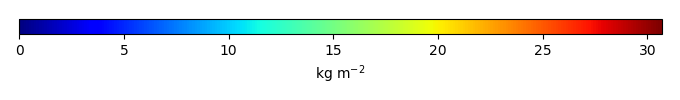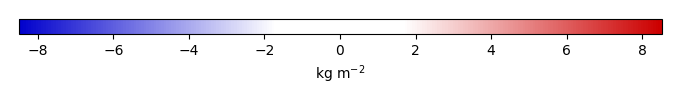# Mean State

Period Mean (original grids) [Pg]
Model Period Mean (intersection) [Pg]
Model Period Mean (complement) [Pg]
Benchmark Period Mean (intersection) [Pg]
Benchmark Period Mean (complement) [Pg]
Bias [kg m-2]
Bias Score 
Spatial Distribution Score 
Overall Score 
Benchmark [-] 32.8
CLM4 [-] 49.5 50.3 0.182 32.8 0.0526 4.47 0.57 0.76 0.67
CLM4.5 [-] 45.4 45.4 0.235 32.8 0.0526 3.37 0.54 0.71 0.63
CLM5 [-] 38.5 38.7 0.241 32.8 0.0526 1.73 0.72 0.93 0.82
Period Mean (original grids) [Pg]
Model Period Mean (intersection) [Pg]
Model Period Mean (complement) [Pg]
Benchmark Period Mean (intersection) [Pg]
Benchmark Period Mean (complement) [Pg]
Bias [kg m-2]
Bias Score 
Spatial Distribution Score 
Overall Score 
Benchmark [-] 8.35
CLM4 [-] 7.61 6.74 0.0800 8.34 0.0114 -0.268 0.63 0.91 0.77
CLM4.5 [-] 2.26 1.89 0.152 8.34 0.0114 -1.12 0.44 0.40 0.42
CLM5 [-] 3.24 2.69 0.201 8.34 0.0114 -0.977 0.48 0.62 0.55
Period Mean (original grids) [Pg]
Model Period Mean (intersection) [Pg]
Model Period Mean (complement) [Pg]
Benchmark Period Mean (intersection) [Pg]
Benchmark Period Mean (complement) [Pg]
Bias [kg m-2]
Bias Score 
Spatial Distribution Score 
Overall Score 
Benchmark [-] 10.6
CLM4 [-] 10.9 10.4 0.214 10.6 0.0246 0.0746 0.55 0.64 0.59
CLM4.5 [-] 4.95 4.63 0.213 10.6 0.0246 -0.565 0.46 0.69 0.57
CLM5 [-] 12.3 11.7 0.363 10.6 0.0246 0.299 0.50 0.56 0.53
Period Mean (original grids) [Pg]
Model Period Mean (intersection) [Pg]
Model Period Mean (complement) [Pg]
Benchmark Period Mean (intersection) [Pg]
Benchmark Period Mean (complement) [Pg]
Bias [kg m-2]
Bias Score 
Spatial Distribution Score 
Overall Score 
Benchmark [-] 4.69
CLM4 [-] 0.798 0.806 0.0186 4.67 0.0241 -1.12 0.44 0.26 0.35
CLM4.5 [-] 3.11 3.09 0.0372 4.67 0.0241 -0.401 0.61 0.68 0.64
CLM5 [-] 4.17 4.12 0.0636 4.67 0.0241 -0.0436 0.58 0.80 0.69
Period Mean (original grids) [Pg]
Model Period Mean (intersection) [Pg]
Model Period Mean (complement) [Pg]
Benchmark Period Mean (intersection) [Pg]
Benchmark Period Mean (complement) [Pg]
Bias [kg m-2]
Bias Score 
Spatial Distribution Score 
Overall Score 
Benchmark [-] 27.8
CLM4 [-] 62.8 60.6 1.99 26.8 0.921 15.4 0.31 0.63 0.47
CLM4.5 [-] 54.1 52.1 1.81 26.8 0.921 13.0 0.39 0.82 0.61
CLM5 [-] 39.9 38.2 1.51 26.8 0.921 7.87 0.61 0.92 0.76
Period Mean (original grids) [Pg]
Model Period Mean (intersection) [Pg]
Model Period Mean (complement) [Pg]
Benchmark Period Mean (intersection) [Pg]
Benchmark Period Mean (complement) [Pg]
Bias [kg m-2]
Bias Score 
Spatial Distribution Score 
Overall Score 
Benchmark [-] 43.5
CLM4 [-] 26.8 26.1 0.0959 43.5 0.0288 -1.84 0.59 0.86 0.72
CLM4.5 [-] 31.7 31.0 0.174 43.5 0.0288 -1.27 0.59 0.86 0.72
CLM5 [-] 52.5 51.4 0.326 43.5 0.0288 1.03 0.49 0.63 0.56
Period Mean (original grids) [Pg]
Model Period Mean (intersection) [Pg]
Model Period Mean (complement) [Pg]
Benchmark Period Mean (intersection) [Pg]
Benchmark Period Mean (complement) [Pg]
Bias [kg m-2]
Bias Score 
Spatial Distribution Score 
Overall Score 
Benchmark [-] 32.0
CLM4 [-] 40.6 40.6 0.605 31.9 0.0804 0.794 0.56 0.77 0.67
CLM4.5 [-] 40.9 40.7 0.650 31.9 0.0804 0.833 0.52 0.74 0.63
CLM5 [-] 31.6 31.8 0.632 31.9 0.0804 0.262 0.61 0.86 0.73
Period Mean (original grids) [Pg]
Model Period Mean (intersection) [Pg]
Model Period Mean (complement) [Pg]
Benchmark Period Mean (intersection) [Pg]
Benchmark Period Mean (complement) [Pg]
Bias [kg m-2]
Bias Score 
Spatial Distribution Score 
Overall Score 
Benchmark [-] 21.1
CLM4 [-] 28.1 26.8 0.449 20.9 0.146 1.69 0.56 0.73 0.65
CLM4.5 [-] 22.9 21.6 0.529 20.9 0.146 1.10 0.49 0.66 0.57
CLM5 [-] 24.8 23.3 0.610 20.9 0.146 1.28 0.60 0.82 0.71
Period Mean (original grids) [Pg]
Model Period Mean (intersection) [Pg]
Model Period Mean (complement) [Pg]
Benchmark Period Mean (intersection) [Pg]
Benchmark Period Mean (complement) [Pg]
Bias [kg m-2]
Bias Score 
Spatial Distribution Score 
Overall Score 
Benchmark [-] 3.81
CLM4 [-] 0.743 0.815 0.0110 3.81 0.000319 -0.856 0.45 0.29 0.37
CLM4.5 [-] 3.24 3.34 0.0509 3.81 0.000319 -0.123 0.63 0.70 0.66
CLM5 [-] 6.88 7.17 0.0941 3.81 0.000319 1.03 0.51 0.75 0.63
Period Mean (original grids) [Pg]
Model Period Mean (intersection) [Pg]
Model Period Mean (complement) [Pg]
Benchmark Period Mean (intersection) [Pg]
Benchmark Period Mean (complement) [Pg]
Bias [kg m-2]
Bias Score 
Spatial Distribution Score 
Overall Score 
Benchmark [-] 9.16
CLM4 [-] 7.63 7.09 0.450 8.87 0.289 0.206 0.57 0.82 0.70
CLM4.5 [-] 7.65 7.05 0.527 8.87 0.289 0.239 0.58 0.84 0.71
CLM5 [-] 11.0 10.0 0.829 8.87 0.289 0.760 0.60 0.81 0.71
Period Mean (original grids) [Pg]
Model Period Mean (intersection) [Pg]
Model Period Mean (complement) [Pg]
Benchmark Period Mean (intersection) [Pg]
Benchmark Period Mean (complement) [Pg]
Bias [kg m-2]
Bias Score 
Spatial Distribution Score 
Overall Score 
Benchmark [-] 12.0
CLM4 [-] 5.40 5.21 0.116 12.0 0.0529 -0.977 0.54 0.67 0.60
CLM4.5 [-] 11.2 10.8 0.257 12.0 0.0529 0.212 0.63 0.85 0.74
CLM5 [-] 18.2 17.4 0.537 12.0 0.0529 1.77 0.47 0.35 0.41
Period Mean (original grids) [Pg]
Model Period Mean (intersection) [Pg]
Model Period Mean (complement) [Pg]
Benchmark Period Mean (intersection) [Pg]
Benchmark Period Mean (complement) [Pg]
Bias [kg m-2]
Bias Score 
Spatial Distribution Score 
Overall Score 
Benchmark [-] 368.
CLM4 [-] 467. 460. 6.82 363. 4.64 1.23 0.52 0.64 0.58
CLM4.5 [-] 453. 445. 8.16 363. 4.64 1.17 0.53 0.71 0.62
CLM5 [-] 487. 477. 9.77 363. 4.64 1.46 0.57 0.78 0.68
Period Mean (original grids) [Pg]
Model Period Mean (intersection) [Pg]
Model Period Mean (complement) [Pg]
Benchmark Period Mean (intersection) [Pg]
Benchmark Period Mean (complement) [Pg]
Bias [kg m-2]
Bias Score 
Spatial Distribution Score 
Overall Score 
Benchmark [-] 1.24
CLM4 [-] 1.32 1.18 0.143 1.23 0.00783 0.0265 0.56 0.77 0.66
CLM4.5 [-] 1.34 1.15 0.206 1.23 0.00783 0.0355 0.52 0.65 0.59
CLM5 [-] 1.55 1.22 0.341 1.23 0.00783 0.0659 0.51 0.74 0.62
Period Mean (original grids) [Pg]
Model Period Mean (intersection) [Pg]
Model Period Mean (complement) [Pg]
Benchmark Period Mean (intersection) [Pg]
Benchmark Period Mean (complement) [Pg]
Bias [kg m-2]
Bias Score 
Spatial Distribution Score 
Overall Score 
Benchmark [-] 5.62
CLM4 [-] 3.07 3.02 0.0260 5.59 0.0265 -0.391 0.59 0.70 0.64
CLM4.5 [-] 7.93 7.70 0.111 5.59 0.0265 0.446 0.60 0.87 0.73
CLM5 [-] 14.5 14.1 0.228 5.59 0.0265 1.60 0.34 0.40 0.37
Period Mean (original grids) [Pg]
Model Period Mean (intersection) [Pg]
Model Period Mean (complement) [Pg]
Benchmark Period Mean (intersection) [Pg]
Benchmark Period Mean (complement) [Pg]
Bias [kg m-2]
Bias Score 
Spatial Distribution Score 
Overall Score 
Benchmark [-] 8.21
CLM4 [-] 4.65 4.16 0.219 8.09 0.122 -0.578 0.46 0.54 0.50
CLM4.5 [-] 8.49 7.87 0.366 8.09 0.122 0.956 0.48 0.50 0.49
CLM5 [-] 10.2 9.46 0.474 8.09 0.122 1.74 0.54 0.60 0.57
Period Mean (original grids) [Pg]
Model Period Mean (intersection) [Pg]
Model Period Mean (complement) [Pg]
Benchmark Period Mean (intersection) [Pg]
Benchmark Period Mean (complement) [Pg]
Bias [kg m-2]
Bias Score 
Spatial Distribution Score 
Overall Score 
Benchmark [-] 14.8
CLM4 [-] 13.4 13.1 0.144 14.8 0.0147 -0.146 0.69 0.91 0.80
CLM4.5 [-] 14.7 14.3 0.191 14.8 0.0147 0.212 0.63 0.78 0.71
CLM5 [-] 12.0 11.7 0.182 14.8 0.0147 -0.425 0.65 0.87 0.76
Period Mean (original grids) [Pg]
Model Period Mean (intersection) [Pg]
Model Period Mean (complement) [Pg]
Benchmark Period Mean (intersection) [Pg]
Benchmark Period Mean (complement) [Pg]
Bias [kg m-2]
Bias Score 
Spatial Distribution Score 
Overall Score 
Benchmark [-] 20.1
CLM4 [-] 16.3 16.3 0.135 19.8 0.219 -0.452 0.59 0.78 0.69
CLM4.5 [-] 28.1 28.1 0.187 19.8 0.219 1.88 0.53 0.86 0.69
CLM5 [-] 43.6 43.6 0.247 19.8 0.219 4.91 0.40 0.72 0.56
Period Mean (original grids) [Pg]
Model Period Mean (intersection) [Pg]
Model Period Mean (complement) [Pg]
Benchmark Period Mean (intersection) [Pg]
Benchmark Period Mean (complement) [Pg]
Bias [kg m-2]
Bias Score 
Spatial Distribution Score 
Overall Score 
Benchmark [-] 8.04
CLM4 [-] 4.00 4.04 0.0137 7.99 0.0515 -0.912 0.52 0.28 0.40
CLM4.5 [-] 2.99 3.01 0.0287 7.99 0.0515 -1.13 0.49 0.26 0.37
CLM5 [-] 3.04 3.04 0.0352 7.99 0.0515 -1.10 0.51 0.49 0.50
Period Mean (original grids) [Pg]
Model Period Mean (intersection) [Pg]
Model Period Mean (complement) [Pg]
Benchmark Period Mean (intersection) [Pg]
Benchmark Period Mean (complement) [Pg]
Bias [kg m-2]
Bias Score 
Spatial Distribution Score 
Overall Score 
Benchmark [-] 88.9
CLM4 [-] 159. 158. 0.324 88.8 0.0655 8.54 0.47 0.55 0.51
CLM4.5 [-] 141. 141. 0.389 88.8 0.0655 6.54 0.55 0.74 0.64
CLM5 [-] 129. 128. 0.373 88.8 0.0655 5.00 0.65 0.94 0.80
Period Mean (original grids) [Pg]
Model Period Mean (intersection) [Pg]
Model Period Mean (complement) [Pg]
Benchmark Period Mean (intersection) [Pg]
Benchmark Period Mean (complement) [Pg]
Bias [kg m-2]
Bias Score 
Spatial Distribution Score 
Overall Score 
Benchmark [-] 9.09
CLM4 [-] 17.8 17.9 0.101 9.08 0.0128 1.48 0.40 0.24 0.32
CLM4.5 [-] 13.6 13.5 0.162 9.08 0.0128 0.827 0.47 0.28 0.38
CLM5 [-] 21.8 21.8 0.283 9.08 0.0128 2.13 0.32 0.30 0.31
Period Mean (original grids) [Pg]
Model Period Mean (intersection) [Pg]
Model Period Mean (complement) [Pg]
Benchmark Period Mean (intersection) [Pg]
Benchmark Period Mean (complement) [Pg]
Bias [kg m-2]
Bias Score 
Spatial Distribution Score 
Overall Score 
Benchmark [-] 2.45
CLM4 [-] 4.80 5.05 0.0796 2.40 0.0496 1.35 0.39 0.27 0.33
CLM4.5 [-] 5.01 5.08 0.102 2.40 0.0496 1.50 0.35 0.28 0.32
CLM5 [-] 6.26 6.15 0.140 2.40 0.0496 1.92 0.35 0.32 0.34

# Temporally integrated period mean

BENCHMARK MEANMODEL MEANBIASBIAS SCORESPATIAL TAYLOR DIAGRAMMODEL COLORS# Spatially integrated regional mean

MODEL COLORS# All Models

BenchmarkCLM4CLM4.5CLM5